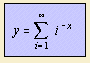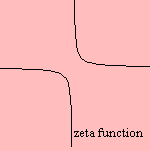# zeta function

## gammaThe zeta function ζ(x) is also named the Riemann zeta function or the Euler zeta function.

For integer values of the argument the zeta function can be found in some sums and integrals.

The zeta function that has been generalized for complex arguments is used to describe the distribution of primes. With its help the Prime Number Theorem can be derived.

The function can be generalized as the generalized zeta function.

The Riemann function can be used to obtain a fairly good approximation of the prime counting function.